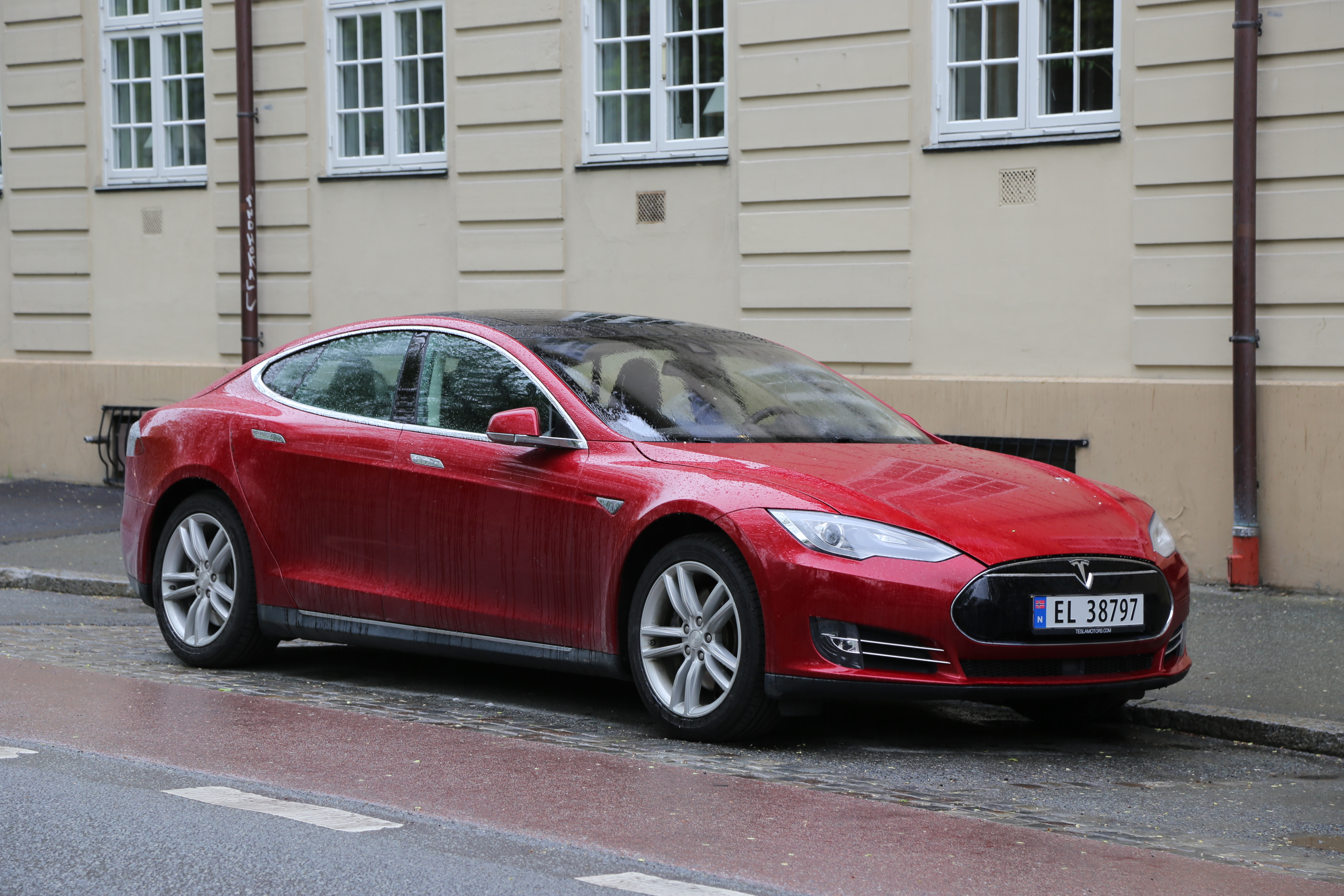# Prediction Classes¶ImageAI provides very powerful yet easy to use classes to perform Image Recognition tasks. You can perform all of these state-of-the-art computer vision tasks with python code that ranges between just 5 lines to 12 lines. Once you have Python, other dependencies and ImageAI installed on your computer system, there is no limit to the incredible applications you can create. Find below the classes and their respective functions available for you to use. These classes can be integrated into any traditional python program you are developing, be it a website, Windows/Linux/MacOS application or a system that supports or part of a Local-Area-Network.

======= imageai.Classification.ImageClassification =======

The ImageClassification class provides you the functions to use state-of-the-art image recognition models like MobileNetV2, ResNet50, InceptionV3 and DenseNet121 that were pre-trained on the the ImageNet-1000 dataset.This means you can use this class to predict/recognize 1000 different objects in any image or number of images. To initiate the class in your code, you will create a new instance of the class in your code as seen below

```from imageai.Classification import ImageClassification
prediction = ImageClassification()
```

We have provided pre-trained MobileNetV2, ResNet50, InceptionV3 and DenseNet121 image recognition models which you use with your ImageClassification class to recognize images. Find below the link to download the pre-trained models. You can download the model you want to use.

After creating a new instance of the ImageClassification class, you can use the functions below to set your instance property and start recognizing objects in images.

• .setModelTypeAsMobileNetV2() , This function sets the model type of the image recognition instance you created to the MobileNetV2 model, which means you will be performing your image prediction tasks using the pre-trained “MobileNetV2” model you downloaded from the links above. Find example code below

```prediction.setModelTypeAsMobileNetV2()
```
• .setModelTypeAsResNet50() , This function sets the model type of the image recognition instance you created to the ResNet50 model, which means you will be performing your image prediction tasks using the pre-trained “ResNet50” model you downloaded from the links above. Find example code below

```prediction.setModelTypeAsResNet50()
```
• .setModelTypeAsInceptionV3() , This function sets the model type of the image recognition instance you created to the InceptionV3 model, which means you will be performing your image prediction tasks using the pre-trained “InceptionV3” model you downloaded from the links above. Find example code below

```prediction.setModelTypeAsInceptionV3()
```
• .setModelTypeAsDenseNet121() , This function sets the model type of the image recognition instance you created to the DenseNet121 model, which means you will be performing your image prediction tasks using the pre-trained “DenseNet121” model you downloaded from the links above. Find example code below

```prediction.setModelTypeAsDenseNet121()
```
• .setModelPath() , This function accepts a string which must be the path to the model file you downloaded and must corresponds to the model type you set for your image prediction instance. Find example code,and parameters of the function below

```prediction.setModelPath("resnet50-19c8e357.pth")
```
• .loadModel() , This function loads the model from the path you specified in the function call above into your image prediction instance. Find example code below

```prediction.loadModel()
```
• .classifyImage() , This is the function that performs actual classification of an image. It can be called many times on many images once the model as been loaded into your prediction instance. Find example code,parameters of the function and returned values below

```predictions, probabilities = prediction.classifyImage("image1.jpg", result_count=10)
```

parameter image_input (required) : This refers to the path to your image file, Numpy array of your image or image file stream of your image, depending on the input type you specified.

parameter result_count (optional) : This refers to the number of possible predictions that should be returned. The parameter is set to 5 by default.

returns prediction_results (a python list) : The first value returned by the predictImage function is a list that contains all the possible prediction results. The results are arranged in descending order of the percentage probability.

returns prediction_probabilities (a python list) : The second value returned by the predictImage function is a list that contains the corresponding percentage probability of all the possible predictions in the prediction_results.

• .useCPU() , This function loads the model in CPU and forces processes to be done on the CPU. This is because by default, ImageAI will use GPU/CUDA if available else default to CPU. Find example code:

```prediction.useCPU()
```

Sample Codes

Find below sample code for predicting one image

```from imageai.Classification import ImageClassification
import os

execution_path = os.getcwd()

prediction = ImageClassification()
prediction.setModelTypeAsResNet50()
prediction.setModelPath(os.path.join(execution_path, "resnet50-19c8e357.pth"))